In Part 12, let’s see how to create histograms in R. Let’s create a simple histogram using the `hist()` command, which is easy to use, but actually quite sophisticated. First, we set up a vector of numbers and then we create a histogram.
```B <- c(2, 4, 5, 7, 12, 14, 16) hist(B)```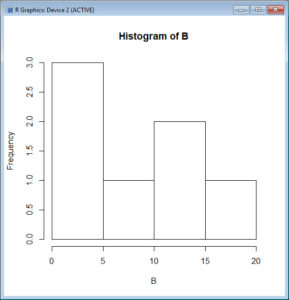That was easy, but you need more from your histogram. Note that R decided on an appropriate bin width. OK. Now we create a histogram from all the data in an array.
```A <- structure(list(James = c(1L, 3L, 6L, 4L, 9L), Robert = c(2L, 5L, 4L, 5L, 12L), David = c(4L, 4L, 6L, 6L, 16L), Anne = c(3L, 5L, 6L, 7L, 6L)), .Names = c("James", "Robert", "David", "Anne"), class = "data.frame", row.names = c(NA, -5L)) attach(A) A```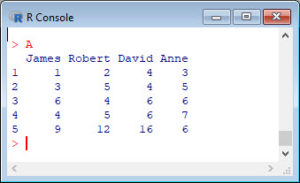The trick is to transform the four variables into a single vector and make a histogram of all elements.
`B <- c(A\$James, A\$Robert, A\$David, A\$Anne)`
Let’s create a histogram of B in dark green and include axis labels.
`hist(B, col="darkgreen", ylim=c(0,10), ylab ="My frequency", xlab ="B values")`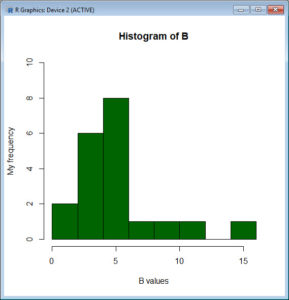However, controlling bin numbers can be problematic. Try setting the number of bins at 6 using the `breaks` argument.
`hist(B, col = "red", breaks=6, xlim=c(0,max(B)), main="My Histogram", las=2, xlab = "B values", cex.lab = 1.3)`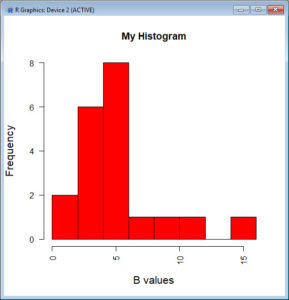You can see that R has taken the number of bins (6) as indicative only. However, setting up histogram bins as a vector gives you more control over the output. Now we set up the bins as a vector, each bin four units wide, and starting at zero.
```bins <- c(0, 4, 8, 12, 16) hist(B, col = "blue", breaks=bins, xlim=c(0,max(B)), main="My Histogram", las=2, xlab = "B values", cex.lab = 1.3)```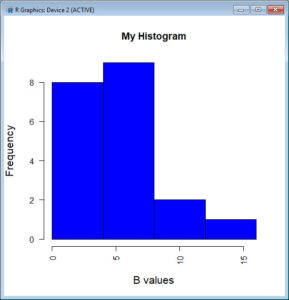Now we have four bins of the right width. That wasn’t so hard! In Blog 13 we will look at further plotting techniques in R.
See you later!
David

#### Annex: R codes used

```# Create a vector of numbers.
B <- c(2, 4, 5, 7, 12, 14, 16)
hist(B)

# Generate a dataset.
A <- structure(list(James = c(1L, 3L, 6L, 4L, 9L), Robert = c(2L, 5L, 4L, 5L, 12L), David = c(4L, 4L, 6L, 6L, 16L), Anne = c(3L, 5L, 6L, 7L, 6L)), .Names = c("James", "Robert", "David", "Anne"), class = "data.frame", row.names = c(NA, -5L))
attach(A)
A

# Transform the four variables into a single vector.
B <- c(A\$James, A\$Robert, A\$David, A\$Anne)

# Make a histogram.
hist(B, col="darkgreen", ylim=c(0,10), ylab ="My frequency", xlab ="B values")

# Make a histogram.
hist(B, col = "red", breaks=6, xlim=c(0,max(B)), main="My Histogram", las=2, xlab = "B values", cex.lab = 1.3)

# Set number of bins and make a histogram.
bins <- c(0, 4, 8, 12, 16)
hist(B, col = "blue", breaks=bins, xlim=c(0,max), main="My Histogram", las=2, xlab = "B values", cex.lab = 1.3)
```